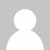Here’s my solutions to day 2 of the Advent of Code challenge, once again using LINQ in C# and then solving in F#:

If you want to see the code. Here’s part a in C#

``````File.ReadAllLines("day2.txt")
.Select(s => s.Split('x'))
.Select(x => x.Select(Int32.Parse))
.Select(w => w.OrderBy(x => x).ToArray())
.Select(w => 3 * w * w + 2 * w * w + 2 * w * w)
.Sum()
``````

Part b in C#

``````File.ReadAllLines("day2.txt")
.Select(s => s.Split('x'))
.Select(x => x.Select(Int32.Parse))
.Select(w => w.OrderBy(x => x).ToArray())
.Select(w => 2 * w + 2 * w + w * w * w)
.Sum()
``````

Part a in F#

``````File.ReadAllLines("day2.txt")
|> Seq.map (fun s -> s.Split('x') |> Seq.map int |> Seq.sort |> Seq.toArray)
|> Seq.map (fun w -> 3 * w. * w. + 2 * w. * w. + 2 * w. * w.)
|> Seq.sum
``````

Part b in F#

``````File.ReadAllLines("day2.txt")
|> Seq.map(fun s->s.Split('x') |> Seq.map int |> Seq.sort |> Seq.toArray)
|> Seq.map(fun w-> 2 * w. + 2 * w. + w. * w. * w.)
|> Seq.sum
``````
Want to learn more about LINQ? Be sure to check out my Pluralsight course LINQ Best Practices.That's nice ; it can be shortened a little though

``Seq.map (fun s -> s.Split 'x' |> Array.map int |> Array.sort)|> Seq.sumBy (fun w -> /* the formula */)// or even|> Seq.sumBy (fun [|a; b; c|] -> /* the formula */)``

Alternatively here is what I've done (note: for each challenge I tried to separate some common code for both parts and just parametrize what is required for each)

``//#nowarn "25" // for incomplete patternlet common =  File.ReadAllLines "day02.txt"  |> Seq.map (fun str ->    str.Split 'x'    |> Array.map int    |> function [|l; w; h|] as arr -> let [|m; n; _|] = Array.sort arr in (l, w, h), (m, n))let part1 () = Seq.sumBy (fun ((l, w, h), (m, n)) -> 2*l*w + 2*l*h + 2*w*h + m*n) commonlet part2 () = Seq.sumBy (fun ((l, w, h), (m, n)) -> l*w*h + 2*m + 2*n) common``

Sehnsuchtnice, yes I should have thought of sumBy, and I'm using a pattern matching a lot more these days but still missing plenty of places I could use it

Mark Heath